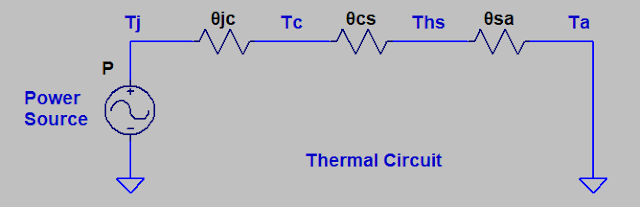# Heat Sink Thermal Resistance Calculator

For calculations of RC Time Constant please visit, RC Time Constant Calculator.

For calculations of Wire Resistance please visit, Wire Resistance Calculator.

Below is the Heat Sink Thermal Resistance Calculator;

 Junction temperature of device: Tj = °C Case temperature of device: Tc = °C Heat sink temperature from datasheet: Ths = °C Ambient temperature: Ta = °C Thermal resistance between junction to case: θjc = °C/W Thermal resistance between case to heat sink: θcs = °C/W Thermal resistance between heat sink to ambient: θsa = °C/W Power dissipation of the device: P = W Airflow force factor: F =

To use this Heat Sink Thermal Resistance Calculator, first enter the values of; Tj, Tc, Ths, Ta, P and F in empty cells. Then click on Calculate to get results of θjc, θcs and θsa.

The Airflow force factor F is an air blower that is used to force cold air through the fins of the heat sink. Select F as 1 if you are not using air blower.

### What is thermal resistance of heatsink?

We know that different types of materials conduct heat differently as per their material properties. We can define the thermal resistance as a property of a material which specifies how good this material conducts heat.

If the thermal resistance value is small, then there will be small temperature rise across the material.

It means that low thermal resistance is a sign for an effective working of material. It will help us to select proper material for the heat sink.

### What is a heat sink?

Heatsink is used to keep the temperature of electronic components as low as possible to avoid an extreme temperature rise. This overheating of a component can result in the failure of the system or circuit.

A heatsink with a small thermal resistance efficiently helps to protect electronic components against excessive temperature.

### Thermal resistance calculation for heat sink

Below diagram shows thermal circuit with heat sink. For thermal resistance calculation for heat sink we will follow this diagram.Thermal Circuit

Below equations will help to calculate the thermal resistance of the heat sink.

The thermal resistance is given by;

θja = θjc + θca

Where;
θja = thermal resistance
θjc = thermal resistance between junction and outside case
θca = thermal resistance between case and ambient

Further, we can split the thermal resistance between case and ambient, θca as;

θca = θcs + θsa

Where;
θcs = thermal resistance between case and heat sink
θsa = thermal resistance between heat sink and ambient

Now we can re-write thermal resistance as;

θja = θjc + θcs + θsa

Further we know that;

θja = (Tj – Ta)/Pd

Where;
Tj = Junction temperature of the device
Ta = Ambient temperature of the device
Pd = Power dissipation of the device

Now we can re-write thermal resistance as;

(Tj – Ta)/Pd = θjc + θcs + θsa

We can re-write it as;

θsa = [(Tj – Ta)/Pd] - θjc - θcs

Where;
θsa = thermal resistance between heat sink and ambient

### Conclusion

Thermal resistance calculation of heat sink will help you for the selection of proper heat sink size. Also, it will help in proper working of component i.e. the generated excessive heat will be reduced by heat sink. Proper resistance selection of heat sink will help to increase life of component and circuit.